# Genetic Algorithms

Use ideas from biological evolution to evolve solutions to problems automatically, rather than designing them by hand.

Genetic algorithms recombine candidate solutions to form new candidates

(Reference: "Genetic Algorithms", John H. Holland, Scientific American, July 1992.)

## Main Components of a Genetic Algorithm

• Encoding of candidate solutions in some formal representation, such as bit strings ("chromosomes") .
• A fitness function that maps candidate solutions to real numbers.
• The fitness function defines a fitness landscape over the search space (similar to error landscape over weight space for neural networks)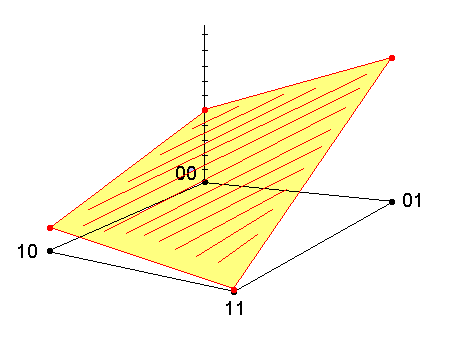f (00) --> 0.5
f (01) --> 0.9
f (10) --> 0.1
f (11) --> 0.0

• A population of candidate solutions
• A set of genetic operators for transforming candidate solutions into new candidate solutions.

## Genetic Operators

• selection - select chromosomes for inclusion in the next generation as a probabilistic function of fitness
• crossover - combine two chromosomes to produce new chromosomes for inclusion in the next generation (akin to sexual recombination in nature)

• mutation - modify components of a chromosome (alleles) with some probability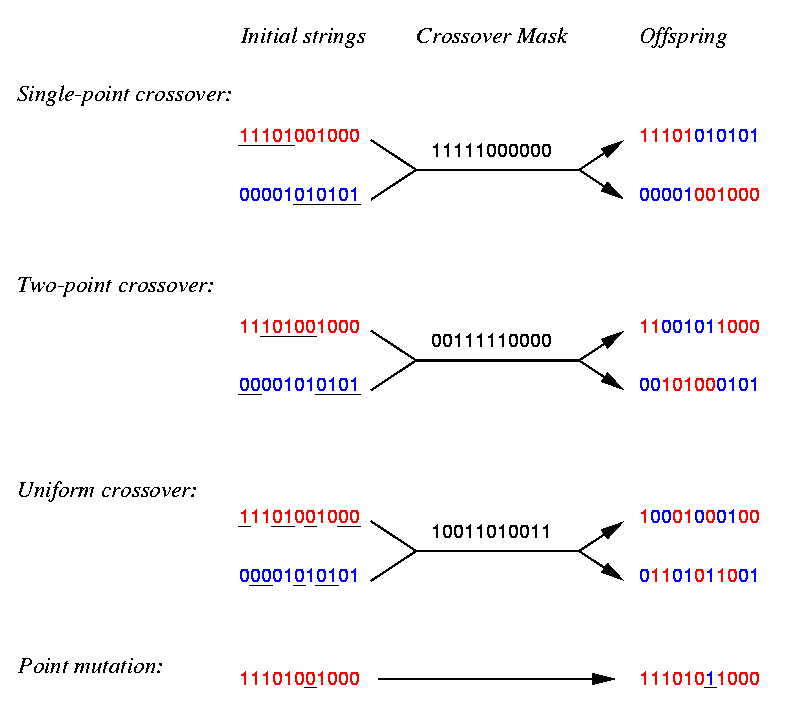## Simple Genetic Algorithm

1. Generate random population of N L-bit chromosomes

2. Calculate fitness f (x) of each chromosome in population

3. Repeat until N offspring have been created:

• Select a pair of chromosomes from the current population as a probabilistic function of fitness
• With probability pc, perform crossover on chromosomes
• Mutate each bit of offspring chromosomes with probability pm
• Add offspring to the new population
4. Replace current population with new population

5. Go to step 2

## Schemas

A schema is a template that represents a set of bit strings

**1**0*  -->  { 1110000, 0010001, 0111001, 0010000, ... }

Every schema s has an estimated average fitness f(s), determined by the fitness function and the current instances of s in the population

Schema Theorem:

Et+1  >   k · [ f(s) / f(pop) ] ·  Et                 (John Holland, 1975)

where

• Et is the expected number of instances of schema s in the population at time t
• f(s) is the estimated average fitness of schema s
• f(pop) is the average fitness of all strings in the population
• k > 0 is a constant that depends on schema s
Schema s receives exponentially increasing or decreasing numbers of instances in the population, depending on ratio f(s) / f(pop)

Above average schemas will tend to spread through population, below-average schemas will tend to disappear

This happens simultaneously for all schemas present in the population ("implicit parallelism")

## Example

• 8-bit chromosomes
• Fitness function f (x) = number of 1 bits in chromosome
• Population size N = 4
• Crossover probability pc = 0.7
• Mutation probability pm = 0.001
 Chromosome Fitness A: 00000110 2 B: 11101110 6 C: 00100000 1 D: 00110100 3

Average fitness of population = 12/4 = 3.0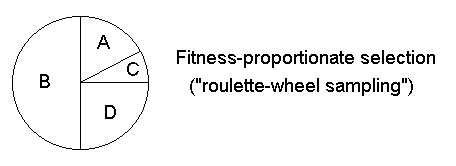1. B and C selected, crossover not performed

2. B mutated

B: 11101110       ---->      B':  01101110

3. B and D selected, crossover performed

B:  11101110                     E:  10110100
---->
D:  00110100                     F:  01101110

4. E mutated

E: 10110100       ---->       E':  10110000

New population:

 Chromosome Fitness B': 01101110 5 C: 00100000 1 E': 10110000 3 F:  01101110 5

Best-fit string from previous population lost, but...

Average fitness of population now 14/4 = 3.5

## Evolving Neural Network Architectures

• Many ways to encode a neural network as a chromosome

• Specificity of genotype->phenotype mapping can vary over a wide range:
• Chromosome encodes each weight value directly
• Chromosome encodes constraints for each weight value (e.g., learnable, fixed, positive, negative)
• Chromosome encodes connectivity between groups of units (e.g., layers, modules)
• Chromosome encodes a genetic "blueprint" that must be translated into a network via a developmental process (which itself may be encoded on the chromosome)

• Example: Miller, Todd, and Hegde evolved network architectures for solving XOR, 4-Quadrant, and Copying problems

• Chromosome specified constraints (learnable vs. fixed) for each weight and bias of the network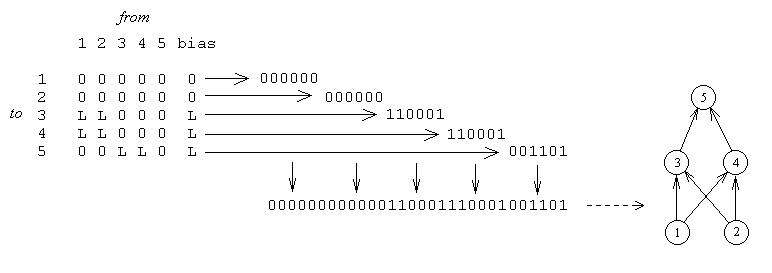• Fitness determined by network's performance on the training task after a fixed number of epochs of backprop with randomly-initialized weights

• Results: GA tended to find asymmetric, non-uniform architectures that worked well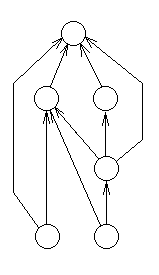## Evolving a Neural Controller for a Simulated Walking Insect

Work by Randy Beer at Case Western Reserve

Reference:  Beer, R.D. (1995), "A dynamical systems perspective on autonomous agents", Artificial Intelligence 72, 173-215.

• simulated insect with six legs ("hexapod")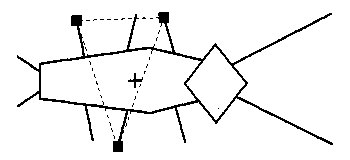• each leg controlled by three effectors ("muscles")
• leg position (up or down)
• forward torque
• backward torque
• at least three legs must be down simultaneously to maintain balance
• center of gravity must lie within polygon spanned by supporting feet
• each leg has a sensor that measures its angle relative to the body axis
• genetic algorithm was used to evolve a neural network for controlling the insect

## Architecture of the Neural Network Controller

• each leg controlled by a fully-recurrent neural network with five units
• continuous-valued units with sigmoid activation functions
• each unit received input from the leg's angle sensor
• three units controlled the leg's effectors
• activation of foot unit determined whether foot was up or down ( > 0.5  ==> up)
• activations of forward/backward swing units determined amount of torque applied
• each leg controller network consisted of 40 parameters:
• 25 recurrent weights
• 5 angle-sensor weights
• 5 biases
• 5 time constants (determined slope of sigmoid)
• complete architecture consisted of six leg-controller networks with identical weights
• ipsilateral (side) connection weights were identical on both sides
• contralateral connection weights (connecting both sides) were identical for all leg pairs
• total of 50 independent parameters to be optimized

## Genetic Algorithm

• each network parameter encoded as four bits
• fitness-proportionate selection (roulette wheel)
• crossover rate 0.6
• mutation rate 0.0001
• population size 500
• fitness function: distance insect moved forward in a fixed amount of time
• evolved for 100 generations

## Problem Requirements

• network has to generate motor signals that allow insect to walk
• insect has to maintain center of gravity

## Results

• early networks stood on all six feet and pushed until they fell
• other networks could move forward but lost their balance
• later networks could move forward while maintaining stability
• some evolved a tripod gait (front and back legs on one side synchronized with opposite middle leg)
• tripod gait is common among fast-walking insects in nature
• no learning algorithm involved -- GA determined neural network weights
• very computationally intensive
• much knowledge is designed in from the start
• body shape
• sensors
• neural network architecture
• training a fully-recurrent neural network is a hard task, but the GA performed well